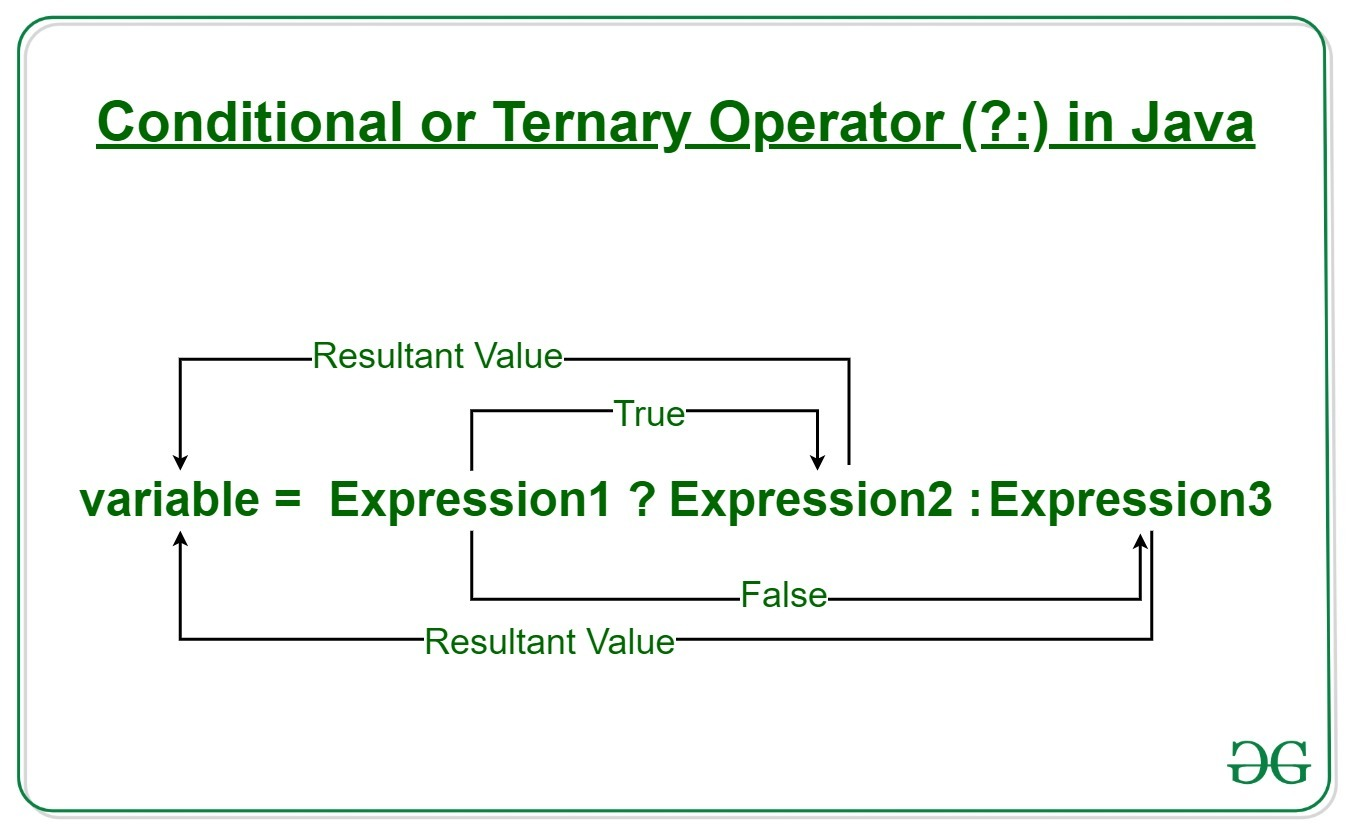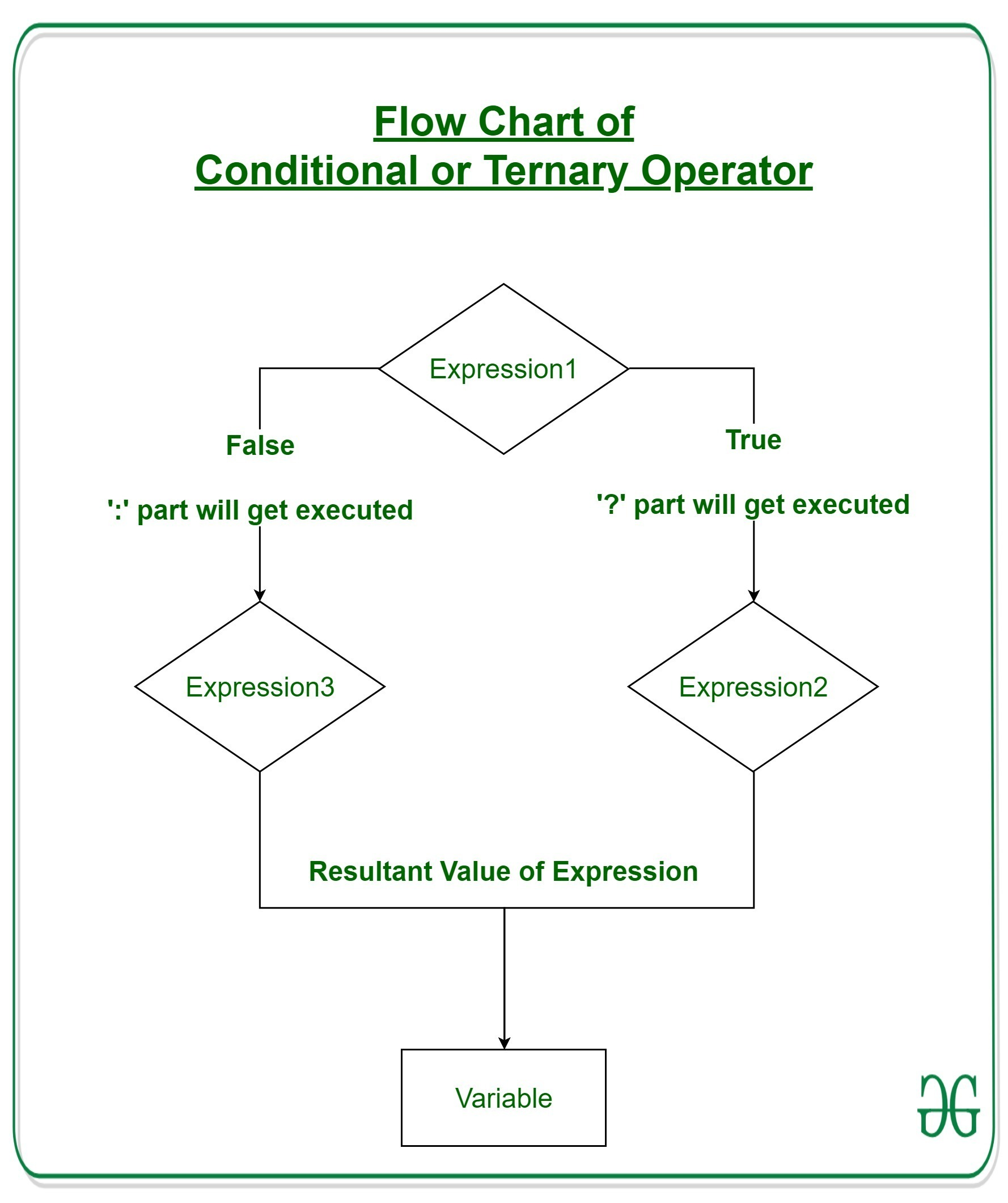Open In App
Related Articles
• Write an Interview Experience
• Java Tutorial
• Java Collection Tutorial

# Java Ternary Operator with Examples

Operators constitute the basic building block to any programming language. Java too provides many types of operators which can be used according to the need to perform various calculations and functions, be it logical, arithmetic, relational, etc. They are classified based on the functionality they provide. Here are a few types:

This article explains all that one needs to know regarding Arithmetic Operators.

### Ternary Operator

Java ternary operator is the only conditional operator that takes three operands. It’s a one-liner replacement for the if-then-else statement and is used a lot in Java programming. We can use the ternary operator in place of if-else conditions or even switch conditions using nested ternary operators. Although it follows the same algorithm as of if-else statement, the conditional operator takes less space and helps to write the if-else statements in the shortest way possible.Syntax:

`variable = Expression1 ? Expression2: Expression3`

If operates similarly to that of the if-else statement as in Exression2 is executed if Expression1 is true else Expression3 is executed.

```if(Expression1)
{
variable = Expression2;
}
else
{
variable = Expression3;
}```

Example:

```num1 = 10;
num2 = 20;

res=(num1>num2) ? (num1+num2):(num1-num2)

Since num1<num2,
the second operation is performed
res = num1-num2 = -10```

#### Flowchart of Ternary OperationExample 1:

## Java

 `// Java program to find largest among two``// numbers using ternary operator` `import` `java.io.*;` `class` `Ternary {``    ``public` `static` `void` `main(String[] args)``    ``{` `        ``// variable declaration``        ``int` `n1 = ``5``, n2 = ``10``, max;` `        ``System.out.println(``"First num: "` `+ n1);``        ``System.out.println(``"Second num: "` `+ n2);` `        ``// Largest among n1 and n2``        ``max = (n1 > n2) ? n1 : n2;` `        ``// Print the largest number``        ``System.out.println(``"Maximum is = "` `+ max);``    ``}``}`

Output

```First num: 5
Second num: 10
Maximum is = 10```

Time Complexity: O(1)
Auxiliary Space: O(1)

Example 2:

## Java

 `// Java code to illustrate ternary operator` `import` `java.io.*;` `class` `Ternary {``    ``public` `static` `void` `main(String[] args)``    ``{` `        ``// variable declaration``        ``int` `n1 = ``5``, n2 = ``10``, res;` `        ``System.out.println(``"First num: "` `+ n1);``        ``System.out.println(``"Second num: "` `+ n2);` `        ``// Performing ternary operation``        ``res = (n1 > n2) ? (n1 + n2) : (n1 - n2);` `        ``// Print the largest number``        ``System.out.println(``"Result = "` `+ res);``    ``}``}`

Output

```First num: 5
Second num: 10
Result = -5```

Time Complexity: O(1)
Auxiliary Space: O(1)

## Java

 `public` `class` `TernaryOperatorExample {``    ``public` `static` `void` `main(String[] args)``    ``{``        ``boolean` `condition = ``true``;``        ``String result = (condition) ? ``"True"` `: ``"False"``;``        ``System.out.println(result);``    ``}``}`

Output

`True`

#### Explanation –

In this program, a Boolean variable condition is declared and assigned the value true. Then, the ternary operator is used to determine the value of the result string. If the condition is true, the value of result will be “True”, otherwise it will be “False”. Finally, the value of result is printed to the console.

#### Advantages of ternary operator –

• Compactness: The ternary operator allows you to write simple if-else statements in a much more concise way, making the code easier to read and maintain.
• Improved readability: When used correctly, the ternary operator can make the code more readable by making it easier to understand the intent behind the code.
• Increased performance: Since the ternary operator evaluates a single expression instead of executing an entire block of code, it can be faster than an equivalent if-else statement.
• Simplification of nested if-else statements: The ternary operator can simplify complex logic by providing a clean and concise way to perform conditional assignments.
• Easy to debug: If a problem occurs with the code, the ternary operator can make it easier to identify the cause of the problem because it reduces the amount of code that needs to be examined.

It’s worth noting that the ternary operator is not a replacement for all if-else statements. For complex conditions or logic, it’s usually better to use an if-else statement to avoid making the code more difficult to understand.